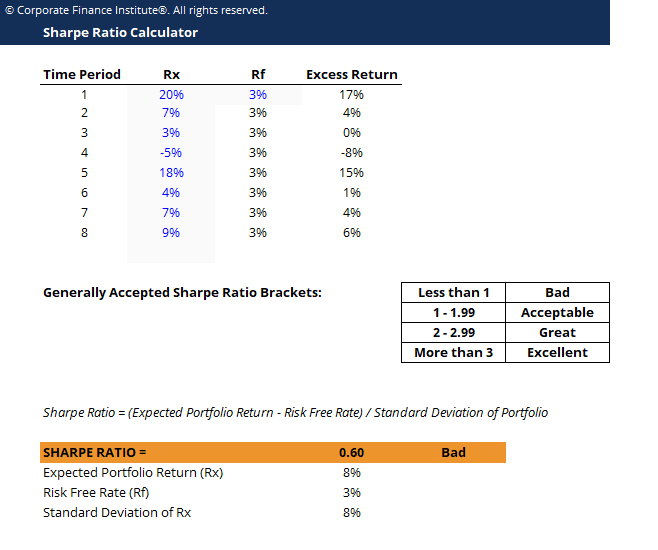# Sharpe Ratio Calculator

## About the Sharpe Ratio Calculator

The Sharpe Ratio, also known as the Sharpe Index, is named after American economist William Sharpe. The ratio is commonly used as a means of calculating the performance of an investment after adjusting for its risk that allows investments of different risk profiles to be compared against each other.

When using the Sharpe Ratio Calculator, a higher value means greater returns for the portfolio relative to the inherent risk, which means a better investment. Because of the simplicity of the formula, the Sharpe Ratio can be used to evaluate a single stock or an entirely diversified portfolio.

### Sharpe Ratio Formula

Sharpe Ratio = (Rx – Rf) / StdDev Rx

Where:

• Rx = Expected portfolio return
• Rf = Risk free rate of return
• StdDev Rx = Standard deviation of portfolio return / volatility

### How to Calculate the Sharpe Ratio in Excel?

Firstly, set up three adjacent columns. The first column should have the header “Time Period”, or something similar, to split the returns into its relevant periods. To the right, the second column should have the header “Portfolio Returns” (Rx). The final column should have the header, “Risk-Free” (Rf).

In the first column, insert the number of relevant periods that there is available portfolio return data for. Optionally, the type of period can be added. For example, if portfolio returns are sorted by years and there are 4 years available, input “Year 1, Year 2, Year 3, and Year 4” into 4 rows within the first column.

In the second column, insert the appropriate portfolio return in percentage for the relevant periods. In the last column, insert the risk-free rate for this particular type of investment or portfolio. The risk-free rate should be the same across all periods.

To calculate the Sharpe Ratio, find the average of the “Portfolio Returns (%)” column using the “=AVERAGE” formula and subtract the risk-free rate out of it. Divide this value by the standard deviation of the portfolio returns, which can be found using the “=STDEV” formula. Alternatively, depending on the version of Excel in use, the formula for standard deviation may be “=STDEVA”.### Sharpe Ratio Calculator

Below are a few general guidelines for evaluating a company’s risk-adjusted return score:

• 2 – 2.99: Great
• Greater than 3: Excellent

While the thresholds are just general guidelines, keep in mind that Sharpe ratios thresholds may differ for investments of particular fields or industries. However, the thresholds are generally accepted, and it is commonly known that any investment or portfolio that returns a Sharpe Ratio of less than 1 is a bad investment or portfolio.

### More Resources

We hope this has been a helpful guide to understanding how to measure risk-adjusted returns with the Sharpe Ratio Calculator. CFI is the official global provider of the Financial Modeling and Valuation Analyst (FMVA)™ certification, designed to transform anyone into a world-class financial analyst.